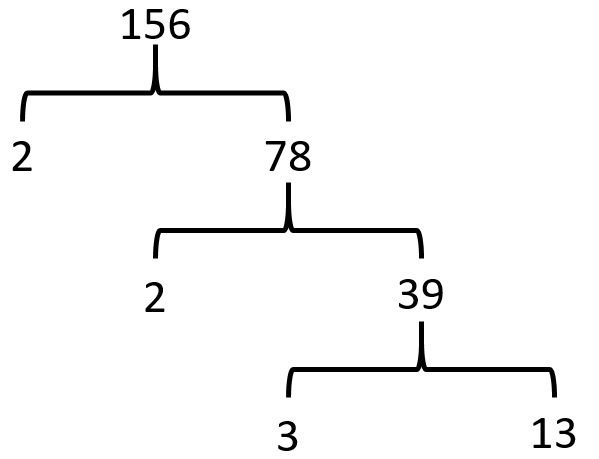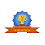# vs.eyeandcontacts.com

## Chapter 1 Real Numbers Exercise 1.2

Question 1: Express each number as a product of its prime factors:
i) 140
ii) 156
iii) 3825
iv) 5005
v) 7429

i) 140ii) 156iii) 3825iv) 5005v) 7429Question 2: Find the LCM and HCF of the following pairs of integers and verify that LCM × HCF = product of the two numbers.
i) 26 and 91
ii) 510 and 92
iii) 336 and 54

i) 26 and 91
Prime factorization of 26 = 2 x 13
Prime factorization of 91 = 7 x 13

Therefore, LCM (26, 91) = 2 x 7 x 13
HCF (26, 91) = 13

Verification:

 Product of 2 numbers HCF x LCM 26 x 91= 2366 182 x 13= 2366

From the above table we can conclude that the LHS side = RHS side or product of 2 numbers = HCF x LCM. Hence verified.

ii) 510 and 92
Prime factorization of 510 = 2 x 3 x 5 x 17
Prime factorization of 92 = 2 x 2 x 23 or 2² x 23

Therefore, LCM (510, 92) = 2² x 3 x 5 x 17 x 23
= 23460
HCF (26, 91) = 2

Verification:

 Product of 2 numbers HCF x LCM 510 x 92= 46920 23460 x 2= 46920

From the above table we can conclude that the LHS side = RHS side or product of 2 numbers = HCF x LCM. Hence verified.

iii) 336 and 54
Prime factorization of 336 = 2 x 2 x 2 x 2 x 3 x 7 or 2⁴ x 3 x 7
Prime factorization of 54 = 2 x 3 x 3 x 3 or 2 x 3³

Therefore, LCM (336, 54) = 2⁴ x 3³ x 7
= 3024
HCF (26, 91) = 2 x 3
= 6

Verification:

 Product of 2 numbers HCF x LCM 336 x 54= 18144 3024 x 6= 18144

From the above table we can conclude that the LHS side = RHS side or product of 2 numbers = HCF x LCM. Hence verified.

Question 3: Find the LCM and HCF of the following integers by applying the prime factorisation method.
i) 12, 15 and 21
ii) 17, 23 and 29
iii) 8, 9 and 25

i) 12, 15 and 21
Prime factorization of 12 = 2 x 2 x 3 or 2² x 3
Prime factorization of 15 = 3 x 5
Prime factorization of 21 = 3 x 7

Therefore, LCM (12, 15, 21) = 2² x 3 x 5 x 7
= LCM = 420
HCF (12, 15, 21) = 3

ii) 17, 23 and 29
Prime factorization of 17 = 1 x 17
Prime factorization of 23 = 1 x 23
Prime factorization of 29 = 1 x 29

Therefore, LCM (17, 23, 29) = 1 x 17 x 23 x 29
= LCM = 11339
HCF (17 , 23, 29) = 1

iii) 8, 9 and 25
Prime factorization of 8 = 2 x 2 x 2 or 2³
Prime factorization of 9 = 3 x 3 or 3²
Prime factorization of 25 = 5 x 5 or 5²

Therefore, LCM (8, 9, 25) = 2³ x 3² x 5²
= LCM = 1800
HCF (8, 9, 25) = 1

Question 4: Given that HCF (306, 657) = 9, find LCM (306, 657).

Given:
HCF of the two numbers = 9
Two numbers = 306 and 657

LCM = ?

We know that,
LCM x HCF = Product of two numbers
= LCM x 9 = 306 x 657
= LCM x 9 = 201,042
= LCM = 201,042/9
= LCM = 22,338

Therefore, LCM (306, 657) = 22,338

Question 5: Check whether 6n can end with the digit 0 for any natural number n.
To end with digit 0, the given number must have factors as 2 and 5. But 6, which has 2 and 3 as factors, has neither 2 nor 5 as factors. Hence, 6 can never end with digit 0.

Question 6: Explain why 7 × 11 × 13 + 13 and 7 × 6 × 5 × 4 × 3 × 2 × 1 + 5 are composite numbers.

For 7 × 11 × 13 + 13,
= 7 x 11 x 13 + 13
= 13 [7 x 11 x 1 + 1]
= 13 [77 + 1]
= 13 
= 13 [2 x 3 x 13]
= 2 x 3 x 13²

Since, 7 x 11 x 13 + 13 can be expressed as a product of primes. Hence, it is a composite number.

For 7 × 6 × 5 x 4 x 3 x 2 x 1 + 5,
= 7 × 6 × 5 x 4 x 3 x 2 x 1 + 5
= 5 [7 × 6 × 5 x 4 x 3 x 2 x 1 + 1]
= 5 [1008 + 1]
= 5 

Since, 7 × 6 × 5 x 4 x 3 x 2 x 1 + 5 can be expressed as a product of primes. Hence, it is a composite number.

Question 7: There is a circular path around a sports field. Sonia takes 18 minutes to drive one round of the field, while Ravi takes 12 minutes for the same. Suppose they both start at the same point and at the same time, and go in the same direction. After how many minutes will they meet again at the starting point?

To find after how many minutes Sonia and Ravi meet again at starting point, we have to find their LCM of their time.

Therefore, LCM (18, 12) = 36

Hence, they will meet after 36 minutes.

#### 1 comment:

1.HomeTop